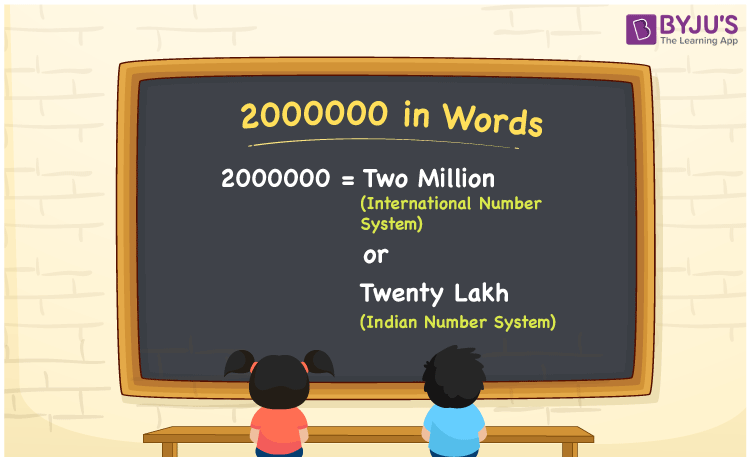# 2000000 in Words

We can write 2000000 in words as Two million in the International number system and as Twenty lakh in the Indian number system. In this article, you will learn how to convert the cardinal number 2000000 to words of both International and Indian number systems.

 2000000 in words Two million (or) Twenty lakh Two million  (or) Twenty lakh in Numbers 2000000

## 2000000 in English Words

We generally write numbers in words using the English alphabet. Thus, we can read 2000000 in English as “Two million” or :Twenty lakh”.## How to Write 2000000 in Words?

2000000 in words – International number system

The below table depicts the place value chart for 2000000 with respect to the International number system.

 Millions Hundred thousands Ten thousands Thousands Hundreds Tens Ones 2 0 0 0 0 0 0

Here, ones = 0, tens = 0, hundreds = 0, thousands = 0, Ten thousands = 0, Hundred thousands = 0, millions = 2

Therefore, 2000000 in words = 2 million = Two million

2000000 in words – Indian number system

Given below is the place value chart for 2000000 in the Indian system.

 Ten lakhs Lakhs Ten thousands Thousands Hundreds Tens Ones 2 0 0 0 0 0 0

Here, ones = 0, tens = 0, hundreds = 0, thousands = 0, ten thousands = 0, lakhs = 0, ten lakhs = 2

That means, 20 lakhs

= Twenty lakhs

2000000 is a natural number that precedes 2000001 and succeeds 1999999.

2000000 in words – Two million (or) Twenty lakhs

Is 2000000 an odd number? – No

Is 2000000 an even number? – Yes

Is 2000000 a perfect square number? – No

Is 2000000 a perfect cube number? – No

Is 2000000 a prime number? – No

Is 2000000 a composite number? – Yes

## Frequently Asked Questions on 2000000 in Words

Q1

### How do you say 2000000 in words?

We can say 2000000 in words as Two million (or) Twenty lakh.
Q2

### How do you write Rs. 2000000 in words for a cheque?

For a cheque, we write Rs. 2000000 in words as “Twenty lakh rupees only”.
Q3

### Is 2000000 the prime number?

No, 2000000 is not a prime number